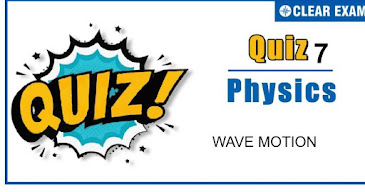##Q1. The frequency of a whistle of an engine is 600 cycles/sec is moving with the speed of 30 m/sec towards an observer. The apparent frequency will be (velocity of sound =330 m/s)
•  330 cps
•  900 cps
•  600 cps
•  660 cps
Solution
n^'=n(v/(v-v_S ))=600(330/300)=660 cps

Q2.The second overtone of an open pipe is in resonance with the first overtone of a closed pipe of length 2m. length of the open pipe is
•  1m
•  4m
•  8m
•  2m
Solution
Second overtone of open pipe of length 𝒍 is v_0=v/2l …….(i) First overtone of a close pipe is v_c=v/4l=v/(4×2)……..(ii) Equating Eqs. (i) and (ii), we get v/2l=v/8⟹l=4m

Q3.  Find the fundamental frequency of a closed pipe, if the length of the air column is 42 m. (speed of sound in air =332 m/sec)
•   9 Hz
•  7 Hz
•  4 Hz
•  2 Hz
Solution
For closed pipe n=v/4l⇒n=332/(4×42)=2Hz

Q4. Speed of sound at constant temperature depends on
•  Pressuree
•  Density of gas
•  Above both
•  None of the above
Solution
Speed of sound, doesn’t depend upon pressure and density medium at constant temperature.

Q5.Out of the given waves (1), (2), (3) and (4) y=a sin⁡〖(kx+ωt)〗 …(1) y=a sin⁡〖(ωt-kx)〗 …(2) y=a cos⁡〖(kx+ωt)〗 …(3) y=a cos⁡〖(ωt-kx)〗 …(4) Emitted by four different sources S_1,S_2,S_3 and S_4 respectively, interference phenomena would be observed in space under appropriate conditions when
•  Source S_2 emits wave (2) and S_4 emits wave (4)
•  S_4 emits waves (4) and S_3 emits waves (3)
•  Source S_3 emits wave (3) and S_4 emits wave (4)
•  Sources S_1 emits wave (1) and S_2 emits wave (2)
Solution
For interference, two waves must have a constant phase relationship. Equation '1' and ‘3’ and ‘2’ and ‘4’ have a constant phase relationship of π/2 out of two choices. Only one S_2 emitting ‘2’ and S_4 emitting ‘4’ is given so only (c) option is correct

Q6. The angle between particle velocity and wave velocity in a transverse wave is
•  π/4
•  π/2
• π
•  0
Solution
Q7.When sound is produced in an aeroplane moving with a velocity of 200 ms^(-1)horizontal its echo is heard after 10√5s. if velocity of sound in air is 300 ms^(-1)the elevation of aircraft is:
•  250 m
•  250 √5 m
•  2500 m
•  12.50 m
Solution
Q8.A wave travelling along a string is described by the equation y=a sin⁡(ωt-kx) the maximum particle velocity is
•  x/l
•
•  ω/k
•  dω/dk
Solution
Given that, the displacement of a particle is Y=a sin (ωt-kx) …(i) The particle velocity v_p=dy/dt …(ii) Now, on differentiating Eq. (i) w.r.t.t, dy/dt=a cos⁡〖(ωt-kx).ω〗 ⟹ dy/dt=aω cos⁡(ωt-kx) From eq. (ii) ⟹ v_p=aω cos⁡(ωt-kx) For maximum particle velocity, cos (ωt-kx)=1 so, v_p=aω×1⟹v_p=aω

Q9.A wave travelling along positive x-axis is given by y=A sin⁡〖(ωt-kx)〗. If it is reflected from rigid boundary such that 80% amplitude is reflected, then equation of reflected wave is
•  y=0.8A sin⁡〖(ωt+kx)〗
•  y=A sin⁡〖(ωt+kx)〗
•  y=A sin⁡〖(ωt+0.8kx)〗
•  y=-0.8A sin⁡〖(ωt+kx)〗
Solution
On getting reflected from a rigid boundary the wave suffers Hence if y_incident=A sin⁡(ωt=kx) Then y_reflected=(0.8A) sin⁡[ωt-k(-x)+π] =-0.8A sin⁡〖(ωt+kx)〗 an additional phase change of π

Q10. A wave travelling in positive X-direction with A=0.2m has a velocity of 360 m/sec. If λ=60m, then correct expression for the wave is
•  y=0.2 sin⁡[2π(6t+x/60)]
•  y=0.2 sin⁡[2π(6t-x/60)]e
•  y=0.2 sin⁡[π(6t-x/60)]
• y=0.2 sin⁡[π(6t+x/60)]
Solution
A wave travelling in positive x-direction may be represented as y=A sin⁡〖2π/λ(vt-x)〗. On putting values y=0.2 sin⁡〖2π/60 (360t-x)⇒y=0.2 sin⁡2π(6t-x/60) 〗#### Written by: AUTHORNAME

AUTHORDESCRIPTION## Want to know more

Please fill in the details below:

## Latest NEET Articles\$type=three\$c=3\$author=hide\$comment=hide\$rm=hide\$date=hide\$snippet=hide

Name

ltr
item
BEST NEET COACHING CENTER | BEST IIT JEE COACHING INSTITUTE | BEST NEET & IIT JEE COACHING: WAVE MOTION QUIZ-7
WAVE MOTION QUIZ-7
https://1.bp.blogspot.com/-qcHcWRLBO_s/X6_kDatDbQI/AAAAAAAANrs/re2KZf_SDi4YjppOqVQCOj6-Acxm3G7ewCLcBGAsYHQ/w365-h204/Copy%2Bof%2BQuiz%2BImage%2BTemplate%2B%25281%2529%2B%252827%2529.jpg
https://1.bp.blogspot.com/-qcHcWRLBO_s/X6_kDatDbQI/AAAAAAAANrs/re2KZf_SDi4YjppOqVQCOj6-Acxm3G7ewCLcBGAsYHQ/s72-w365-c-h204/Copy%2Bof%2BQuiz%2BImage%2BTemplate%2B%25281%2529%2B%252827%2529.jpg
BEST NEET COACHING CENTER | BEST IIT JEE COACHING INSTITUTE | BEST NEET & IIT JEE COACHING
https://www.cleariitmedical.com/2020/11/wave-motion-quiz-7.html
https://www.cleariitmedical.com/
https://www.cleariitmedical.com/
https://www.cleariitmedical.com/2020/11/wave-motion-quiz-7.html
true
7783647550433378923
UTF-8

STAY CONNECTED